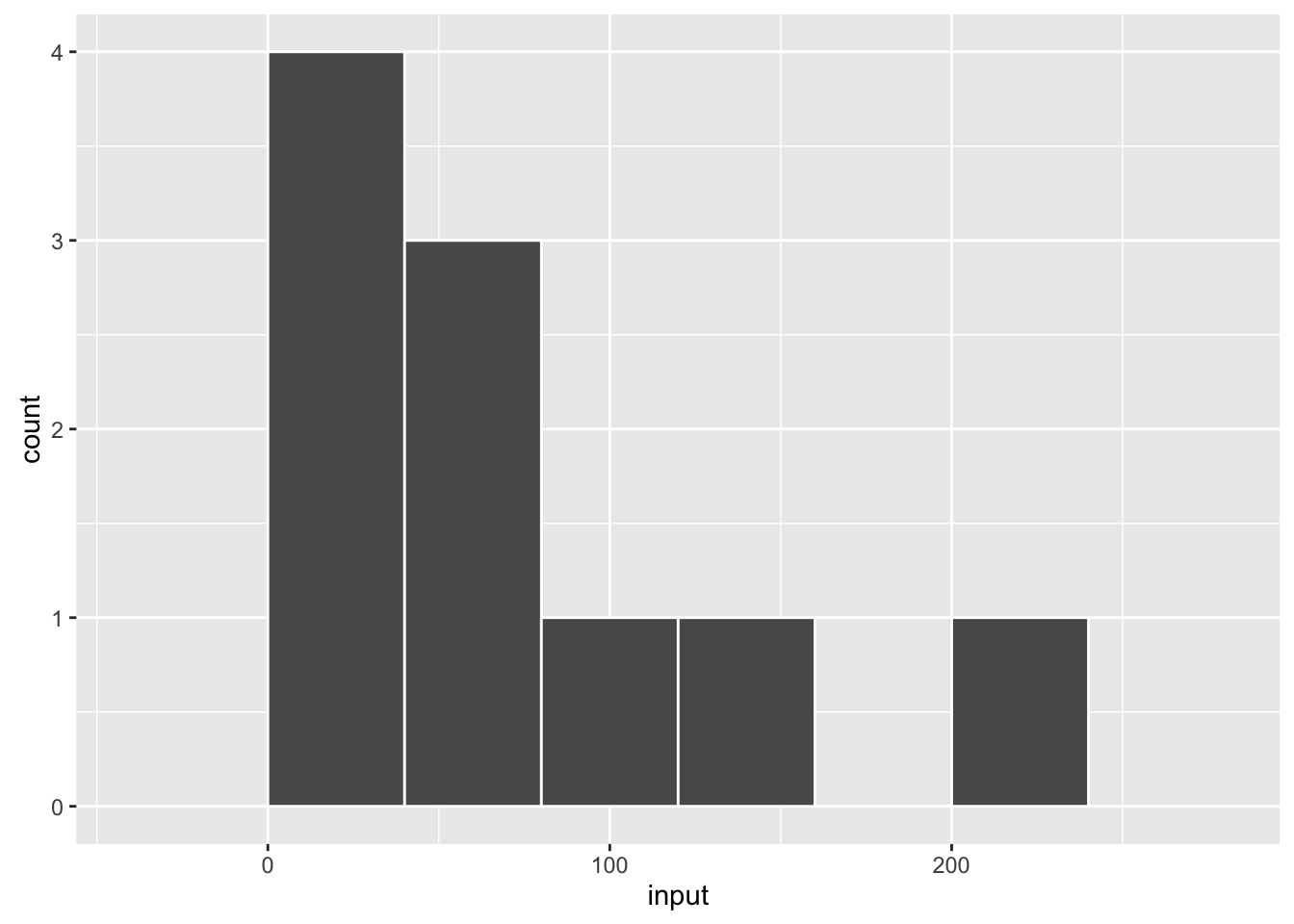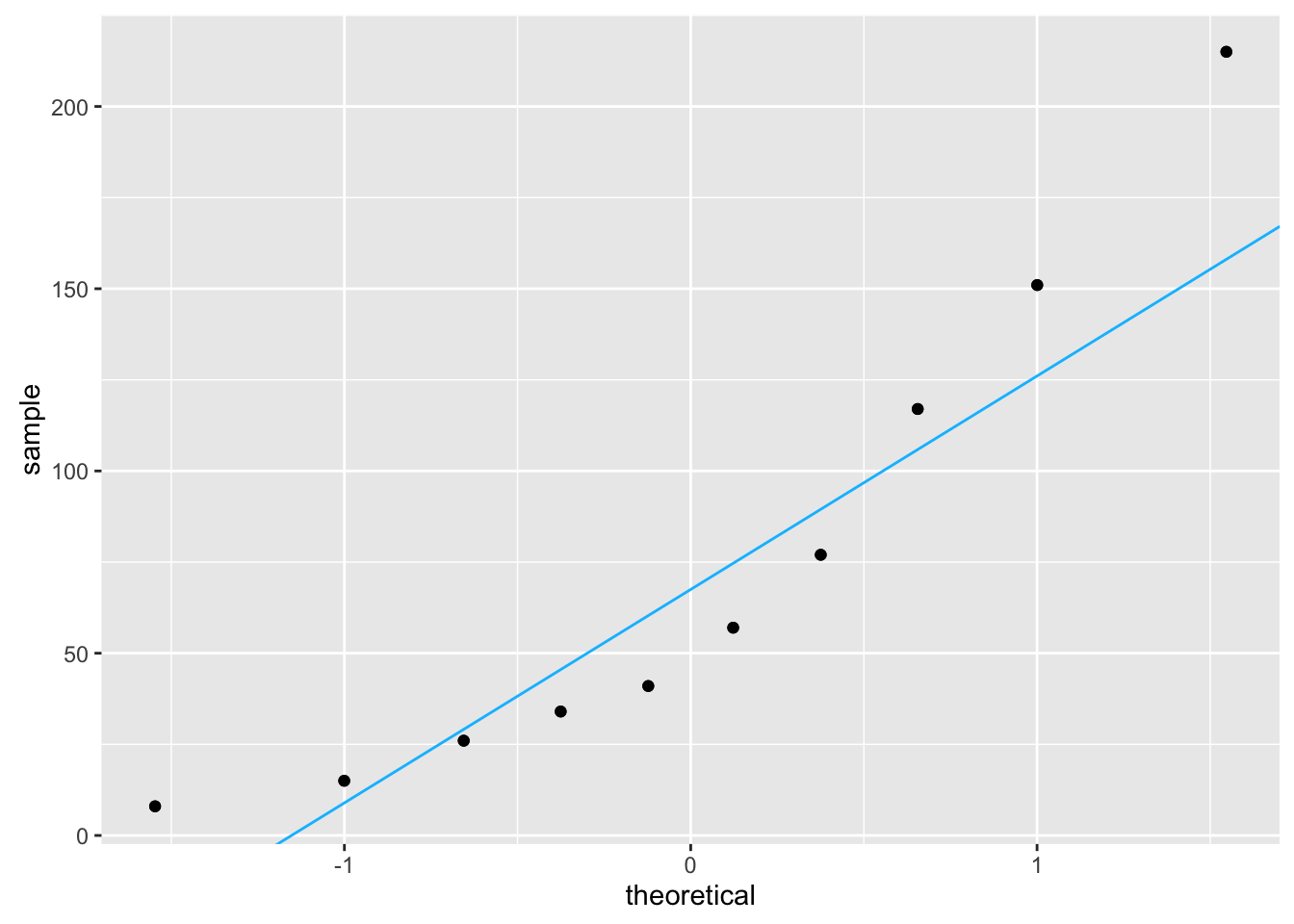# List of useful packages
pkg <- c("ggplot2", "devtools")

# Check if packages are not installed and assign the
# names of the uninstalled packages to the variable new.pkg
new.pkg <- pkg[!(pkg %in% installed.packages())]

# If there are any packages in the list that aren't installed,
# install them
if (length(new.pkg)) {
install.packages(new.pkg, repos = "http://cran.rstudio.com")
}

if(!require(plotly)){
devtools::install_github("ropensci/plotly")
}

library(ggplot2)
library(plotly)

input <- c(57, 15, 41, 117, 26, 151, 8, 77, 215, 34)

## Plot of input

qplot(input, binwidth = 40, col = I("white"))## Q-Q plot to check for normality of input

ggplotly(qplot(sample = input) + stat_qq())

## Function to place line over the top of Q-Q plot representing perfect normal match

qqplot_line <- function(vec) # argument: vector of numbers
{
y <- quantile(vec, c(0.25, 0.75))
x <- qnorm(c(0.25, 0.75))
slope <- diff(y)/diff(x)
int <- y - slope * x

qplot(sample = vec) +
stat_qq() +
geom_abline(slope = slope, intercept = int, col = "deepskyblue")
}

# Call the function with input as the argument

qqplot_line(vec = input)